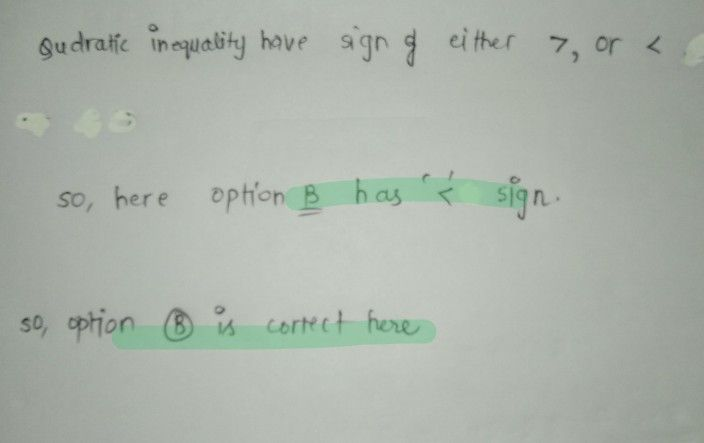Symbol
ProblemDirections. Read each item carefully. Solve if necessary. Write the letter of the correct $7$ $c$ on the space provided before the number. $1$ Which of the following mathematical statements is a quadratic inequality? $C1$ $2t^{2}+8t-9\geq 0$ $0s$ $+8$ $+1540$ $A$ $5r^{2}-4$ $-4r-5=0$ $B$ $3y+12<0$ $-$ $-$
7th-9th grade
Other
Search count: 122
SolutionQanda teacher - Anjali01Option B is correct here
If you have any doubt regarding the solution then please ask